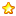# 51单片机定时方式点亮LED灯闪烁C语言程序代码9 |0 | 2021-6-5 01:18:19 | 显示全部楼层 |阅读模式1. 主函数代码如下
2. #include "timer.h"
3. void main()
4. {
5.         unsigned char time = 0;    //定义计数次数的数据类型
6.         P0M0 = 0; P0M1 =1;    //配置P0为准双向模式
7.         Timer0_Init();        //调用初始化函数,初始化定时器0
8.         while(1)
9.         {
10.                 if(TF0 == 1)      //T0溢出标志位，溢出后硬件置 1 （检测定时器是否溢出）
11.                 {
12.                         TF0  = 0;     //软件查询，软件清 0
13.                         time++;       //记录溢出的次数
14.                         if(time == 30)   //0.3s
15.                         {   LED1 = 1;
16.                                 LED2 = 1;
17.                             LED0 = 0;
18.                         }
19.                         if(time == 60)  //0.6s
20.                         {   LED0  = 1;
21.                                 LED2  = 0;
22.                         }
23.                         if(time == 90)  //0.9s
24.                         {   LED2  = 1;
25.                                 LED1  = 0;
26.                                 time  = 0;
27.                         }
28.                 }
29.         }
30. }

31. 有关定时器的点C代码如下
32. #include "timer.h"

33. void Timer0_Init(void)  //溢出定时的时间为10ms
34. {
35.         TMOD = 0X00;   //工作模式寄存器，设置为启动禁止仅由TR0控制，设置为定时模式，工作模式设置为模式0
36.         TH0  = 0XDC;   //给加法计数器附初值 0XDC（65536-9213）/256
37.         TL0  = 0X00;   //                       （65536-9213）%256
38.         TR0  = 1;      //TR0 T0的运行控制位，等于1开始计数
39. }

40. ```
41. 定时器点H代码如下
42. #ifndef __TIMER_H__
43. #define __TIMER_H__

44. #include <STC15W.h>      //15单片机头文件

45.         sbit LED0 = P0^5;    //LED灯的位定义（蓝）
46.         sbit LED1 = P0^6;    // 绿
47.         sbit LED2 = P0^7;    // 红

48. void Timer0_Init(void);

49. #endif

 本版积分规则 回帖后跳转到最后一页302主题 124积分E币
124